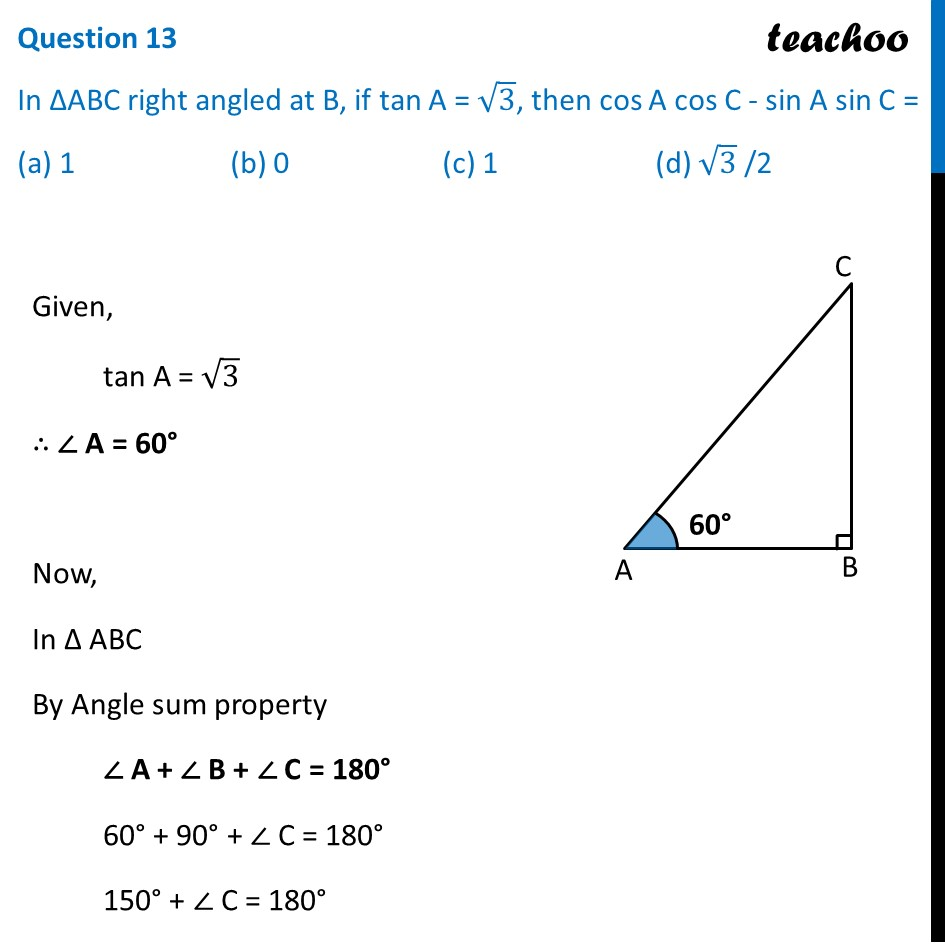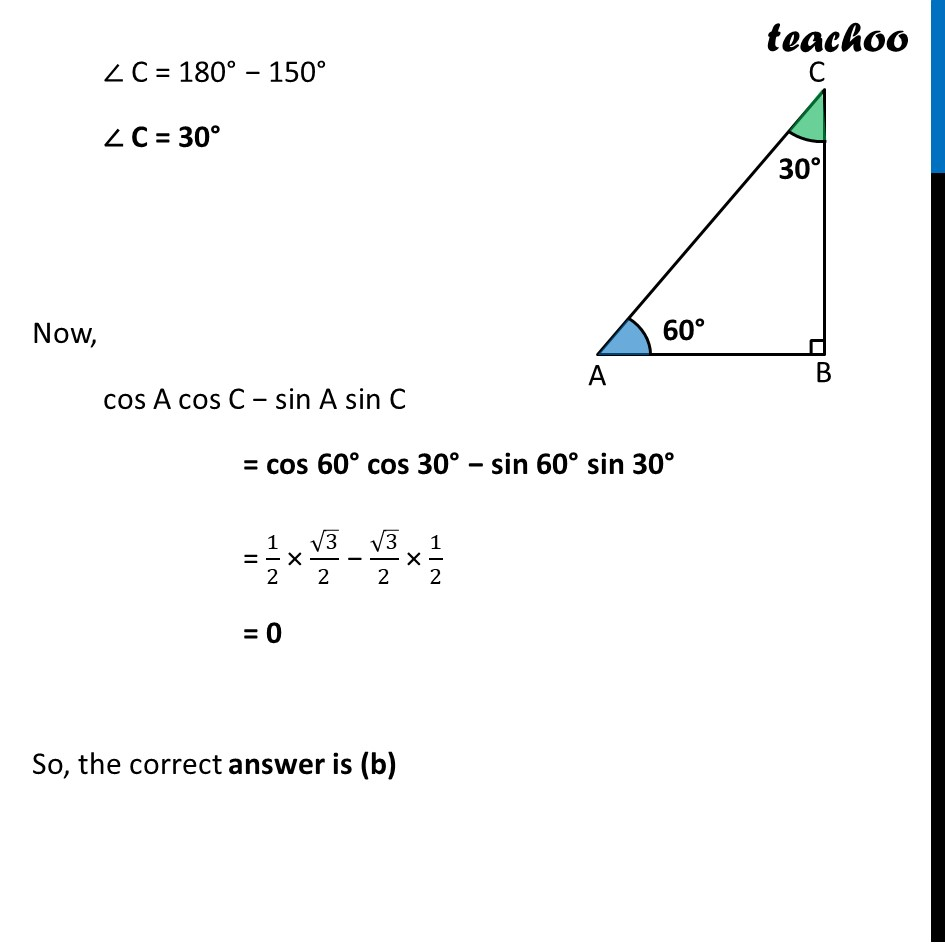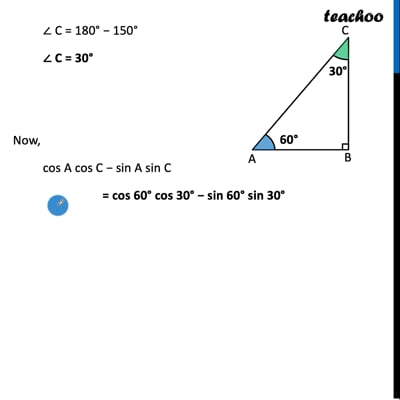CBSE Class 10 Sample Paper for 2022 Boards - Maths Standard [MCQ]

Class 10
Solutions of Sample Papers for Class 10 Boards

## (a) 1   (b) 0   (c) 1   (d) √3  /2

This question is inspired from Ex 8.1, 9 - Chapter 8 Class 10 - Introduction to TrignometryThis video is only available for Teachoo black users

Introducing your new favourite teacher - Teachoo Black, at only ₹83 per month

### Transcript

Question 13 In ∆ABC right angled at B, if tan A = √3, then cos A cos C - sin A sin C = (a) 1 (b) 0 (c) 1 (d) √3 /2 Given, tan A = √3 ∴ ∠ A = 60° Now, In Δ ABC By Angle sum property ∠ A + ∠ B + ∠ C = 180° 60° + 90° + ∠ C = 180° 150° + ∠ C = 180° ∠ C = 180° − 150° ∠ C = 30° Now, cos A cos C − sin A sin C = cos 60° cos 30° − sin 60° sin 30° = 1/2 × √3/2 − √3/2 × 1/2 = 0 So, the correct answer is (b)